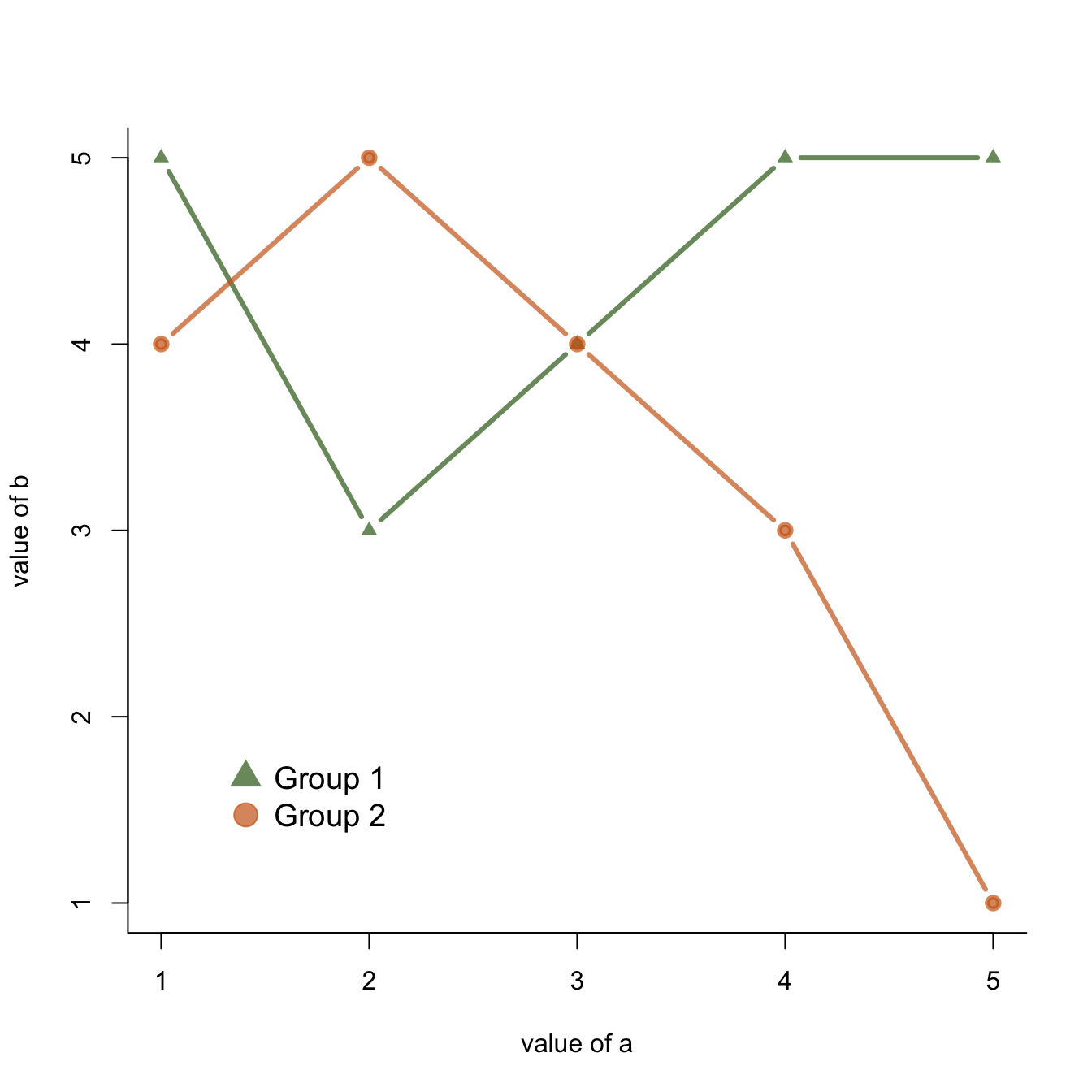# Add a legend to a base R chart

This post explains how to add a legend to a chart made with base R, using the `legend()` function. It provides several reproducible examples with explanation and `R` code.

This page aims to explain how to add a legend to a plot made in base R. It is done using the `legend()` function. The main arguments are:

• `legend` : names to display
• `bty`: type of box around the legend. See graph #73
• `horiz` : legend in column or in row

• `col` : symbol color
• `pch`: symbol type. See graph #6
• `pt.cex` : symbol size

• `cex` : text size
• `text.col`: text color

• `topright` : legend position: `bottomright`, `bottom`, `bottomleft`, `left`, `topleft`, `top`, `topright`, `right`, `center`.
• `inset` : % (from 0 to 1) to draw the legend away from x and y axis
• You can also give the `X` and `Y` coordinate of the legend: `legend(3, 5, ...)`

Note that an equivalent page exist concerning legends with ggplot2.``````# Create data:
a=c(1:5)
b=c(5,3,4,5,5)
c=c(4,5,4,3,1)

# Make a basic graph
plot( b~a , type="b" , bty="l" , xlab="value of a" , ylab="value of b" , col=rgb(0.2,0.4,0.1,0.7) , lwd=3 , pch=17 , ylim=c(1,5) )
lines(c ~a , col=rgb(0.8,0.4,0.1,0.7) , lwd=3 , pch=19 , type="b" )

legend("bottomleft",
legend = c("Group 1", "Group 2"),
col = c(rgb(0.2,0.4,0.1,0.7),
rgb(0.8,0.4,0.1,0.7)),
pch = c(17,19),
bty = "n",
pt.cex = 2,
cex = 1.2,
text.col = "black",
horiz = F ,
inset = c(0.1, 0.1))``````

Related chart types

## Contact

This document is a work by Yan Holtz. Any feedback is highly encouraged. You can fill an issue on Github, drop me a message on Twitter, or send an email pasting yan.holtz.data with gmail.com.